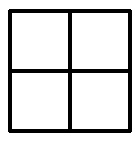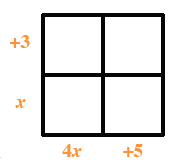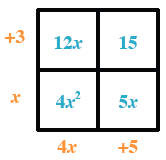### Home > INT1 > Chapter 3 > Lesson 3.3.1 > Problem3-117

3-117.

Multiply each of the following expressions. Show all of your work.

1. $(x+3)(4x+5)$

Use a generic rectangle to multiply.  A 2 by 2 rectangle.

Put the products on the outside of the rectangle. Added to the rectangle, the following labels: left side, top, + 3, left side, bottom, x, bottom side, left, 4, x, bottom side, right, +, 5.

Use length times width to find the areas of the small rectangles. Labels added to the interior of the rectangle as follows: top left, 12 x, top right, 15, bottom left, 4 x squared, bottom right, 5 x.

Write the total area as a sum.
$4x^2+12x+5x+15$

$4x^2+17x+15$1. $(−2x^2−4x)(3x+4)$

Follow the steps in part (a).

$−6x^3−20x^2−16x$

2. $(3y−8)(−x+y)$

Follow the steps in part (a).

3. $(y−4)(3x+5y−2)$

Follow the steps in part (a).